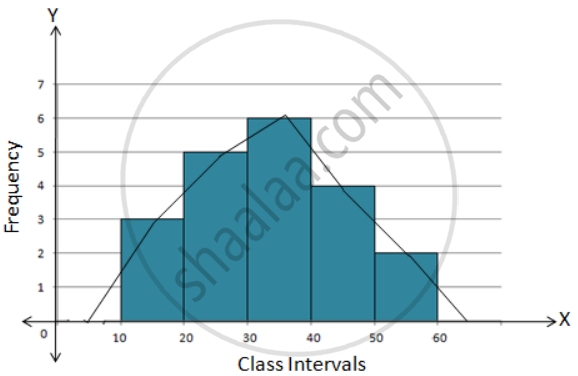# Construct a Combined Histogram and Frequency Polygon for the Following Frequency Distribution: - Mathematics

Graph
Sum

Construct a combined histogram and frequency polygon for the following frequency distribution:

 Class-Intervals 10 - 20 20 - 30 30 - 40 40 - 50 50 - 60 Frequency 3 5 6 4 2

#### Solution

Steps:

1. Draw a histogram for the given data.

2. Mark the mid-point at the top of each rectangle of the histogram drawn.

3. Also, mark the mid-point of the immediately lower class-interval and mid-point of the immediately higher class-interval.

4. Join the consecutive mid-point marked by straight lines to obtain the required frequency polygon.

5. The require combined histogram and frequency polygon are shown in the following figure:Concept: Graphical Representation of Data
Is there an error in this question or solution?

#### APPEARS IN

Selina Concise Mathematics Class 9 ICSE
Chapter 18 Statistics
Exercise 18 (B) | Q 2 | Page 233